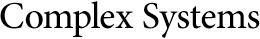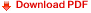## Replication of a Binary Image on a One-Dimensional Cellular Automaton with Linear RulesU Srinivasa Rao*
Jeganathan L

School of Computing Science and Engineering
Vellore Institute of Technology
Vandalur-Kelambakkam Road, Chennai, India-600 127
*umitty.srinivasarao@vit.ac.in
jeganathan.l@vit.ac.in

#### Abstract

A two-state, one-dimensional cellular automaton (1D CA) with uniform linear rules on an (r + 1)-neighborhood replicates any arbitrary binary image given as an initial configuration. By these linear rules, any cell gets updated by an EX-OR operation of the states of extreme (first and last) cells of its (r + 1)-neighborhood. These linear rules replicate the binary image in two ways on the 1D CA: one is without changing the position of the original binary image at time step t = 0 and the other is by changing the position of the original binary image at time step t = 0. Based on the two ways of replication, we have classified the linear rules into three types. In this paper, we have proven that the binary image of size m gets replicated exactly at time step 2k

of the uniform linear rules on the (r + 1)-neighborhood 1D CA, where k is the least positive integer satisfying the inequality m/r less than or equal to 2k. We have also proved that there are exactly (r * 2k - m) cells between the last cell of the binary image and the first cell of the replicated binary image (or the first cell of the binary image and the last cell of the replicated image).

Keywords: replication; binomial coefficients; linear rules; binary image; rule 90